# Math mini-project

## Information

Following is an example of a math mini-project. Students were required to use Scientific Notbebook (or other computer algebra system) to explain an application of differential equations.

Used with permission.

# Newton's Law of Cooling

First_order linear differential equations can be used to solve a variety of problems that involve temperature .For example:

1. a medical examiner can find the time of death in a homicide case,

2. a chemist can determine the time required for a plastic mixture to cool to a hardening temperature

3. and an engineer can design the cooling and heating system of a manufacturing facility although distinct;

Each of these problems depends on a basic principle that is used to develop the associated differential equation, we discuss this important law now.

• basic concept of Newton's law of cooling

Newton's law of cooling states that the rate at which the temperature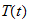changes in a cooling body is proportional to the differences between the temperature of the body and the constant temperature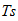of the surrounding medium; this situation is represented as the first_order initial _value problem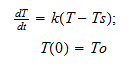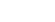Where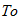is the initial temperature of the body and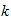is the constant of proportionality; we investigate problems involving Newton's law of cooling in the following examples

In the ideal case , the temperature of the surroundings was assumed to be constant;

However ,this does not have to be the case ;for example, consider the problem of heating and cooling a building ;over the span of a twenty-four hour day, the outside temperature varies ;the problem of determining the temperature inside the building ,therefore ,becomes more complicated ;for the meantime,lets's assume that the building has no heating or air conditioning system ;hence the differential equation that should be solved to find the temperature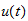at time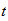inside the building is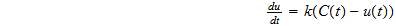Where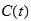is a function that describes the outside temperature and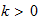is a constant that depends on the insulation of the building; according to this equation ,if,then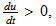which implies that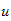increase ;conversely ,if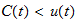,then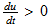,which means thatdecreases.

• Example:

(a) suppose that during the month of April in Atlanta, Georgia, the outside temperature is given by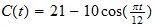,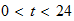;(Note: this implies that the average value ofis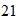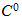) determine the temperature in a building that has an initial temperature of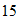if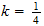;

(b)compare this to the temperature in June when the outside temperature is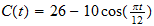and the initial temperature issolution: (a)the initial_value problem that we must solve in April is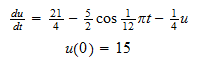, Exact solution is: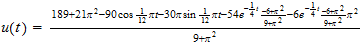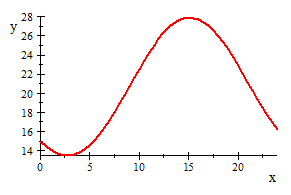Next I find the Max temperature at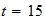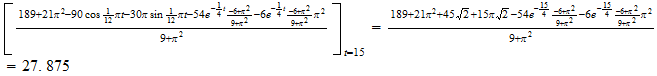From the above calculating and graph ,we can see that the temperature reaches its Max near;and its Max temperature is about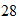(b)solve the temperature in June when the outside temperature isand the initial temperature is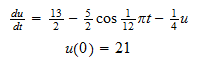, Exact solution is: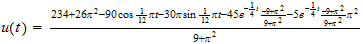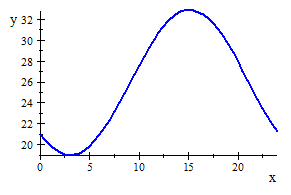Next I find the Max temperature at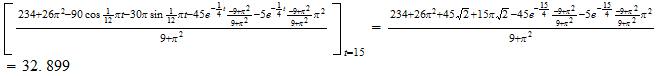from the above calculate and the graph ,we can see that for the June ,its Max temperature is about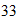Again the Max temperature is also near,and its value is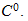• conclusion:

1. From the above comparison ,we can see that during the month of April and June in Atlanta, Georgia, the outside Max temperature time is around;in other words ,around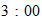pm ,it is hottest; Advice you not to go out at the time;

2. so we can get that first_order linear differential equations can be used to solve a variety of problems that involve temperature ;We can use the basic principle NEWTON'S LAW OF COOLING to find the temperature of the body ,solving some complex practical problems;

3. .It is likely that many of the future application that will best exploit the technological capability of temperature problem have yet to be developed;

• Bibliography

Differential equations with maple

Author is Martha l.abell/james p.braselton

Class no. in library of NP: QA371.5 D37 A141